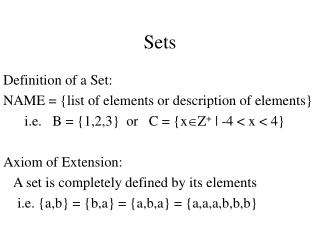# Sets - PowerPoint PPT PresentationDownload PresentationSets

Sets
Download Presentation## Sets

- - - - - - - - - - - - - - - - - - - - - - - - - - - E N D - - - - - - - - - - - - - - - - - - - - - - - - - - -
##### Presentation Transcript

1. Sets Definition of a Set: NAME = {list of elements or description of elements} i.e. B = {1,2,3} or C = {xZ+ | -4 < x < 4} Axiom of Extension: A set is completely defined by its elements i.e. {a,b} = {b,a} = {a,b,a} = {a,a,a,b,b,b}

2. Subset AB  xU, xAxB A is contained in B B contains A A B  x U, xA ^ xB Relationship between membership and subset: xU, xA  {x}  A Definition of set equality: A = B  A B ^ B A

3. Same Set or Not?? X={xZ | p Z, x = 2p} Y={yZ | qZ, y = 2q-2} A={xZ | i Z, x = 2i+1} B={xZ | i Z, x = 3i+1} C={xZ | i Z, x = 4i+1}

4. Set OperationsFormal Definitions and Venn Diagrams Union: Intersection: Complement: Difference:

5. Ordered n-tuple and the Cartesian Product • Ordered n-tuple – takes order and multiplicity into account • (x1,x2,x3,…,xn) • n values • not necessarily distinct • in the order given • (x1,x2,x3,…,xn) = (y1,y2,y3,…,yn)  iZ1in, xi=yi • Cartesian Product

6. Formal Languages •  = alphabet = a finite set of symbols • string over  = empty (or null) string denoted as  OR ordered n-tuple of elements • n = set of strings of length n • * = set of all finite length strings

7. Empty Set Properties • Ø is a subset of every set. • There is only one empty set. • The union of any set with Ø is that set. • The intersection of any set with its own complement is Ø. • The intersection of any set with Ø is Ø. • The Cartesian Product of any set with Ø is Ø. • The complement of the universal set is Ø and the complement of the empty set is the universal set.

8. Other Definitions • Proper Subset • Disjoint Set A and B are disjoint • A and B have no elements in common • xU, xAxB ^ xBx A AB = Ø  A and B are Disjoint Sets • Power Set P(A) = set of all subsets of A

9. Properties of Sets in Theorems 5.2.1 & 5.2.2 • Inclusion • Transitivity • DeMorgan’s for Complement • Distribution of union and intersection

10. Using Venn Diagrams to help find counter example

11. Deriving new Propertiesusing rules and Venn diagrams

12. Partitions of a set • A collection of nonempty sets {A1,A2,…,An} is a partition of the set A if and only if • A = A1A2…An • A1,A2,…,An are mutually disjoint

13. Proofs about Power Sets Power set of A = P(A) = Set of all subsets of A • Prove that A,B {sets}, AB  P(A)  P(B) • Prove that (where n(X) means the size of set X) A {sets}, n(A) = k  n(P(A)) = 2k